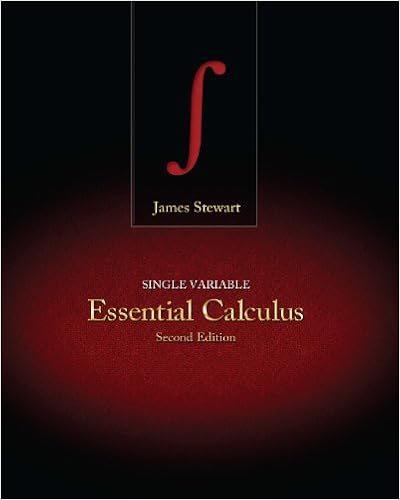# old-exam%281322%29(5) - MAT 1322 SAMPLE EXAMINATION Time 3...

• Notes
• 12

This preview shows page 1 - 4 out of 12 pages.

##### We have textbook solutions for you!
The document you are viewing contains questions related to this textbook.The document you are viewing contains questions related to this textbook.
Chapter 7 / Exercise 22
Single Variable Essential Calculus
StewartExpert Verified
MAT 1322SAMPLE EXAMINATIONTime: 3 hoursCalculators are permitted. (Non-programmable, non-graphing, no differentiation or in-tegration capability.)Notes or books are not permitted.Work the problems in the space provided. Use the back-pages for rough work if necessary.Do not use any other paper. Show all work.Circle the correct answers for multiple choice problems. Numerical answers are rounded.Work on multiple choice problems will be examined in case of suspected fraud.
##### We have textbook solutions for you!
The document you are viewing contains questions related to this textbook.The document you are viewing contains questions related to this textbook.
Chapter 7 / Exercise 22
Single Variable Essential Calculus
StewartExpert Verified
1. [4 points]The finite region bounded by curvesy=x2andy= 4 is rotated about theliney=-2. Sketch the region. Find the volume of the resulting solid.Work.
2.[4 points] An object is taken from an oven at a temperature of 300˚C to a roomat 20˚C. Its temperatureu=u(t) then decreases according to Newton’s law of coolingdudt=k(u-20). After 15 minutes its temperature is 200˚C.(a)Find the temperatureu(t) =(b)When will the temperature reach 100˚C?t=(c)Sketch the graph ofu(t) showing the values fort= 0 and ast→ ∞.Work.
•••